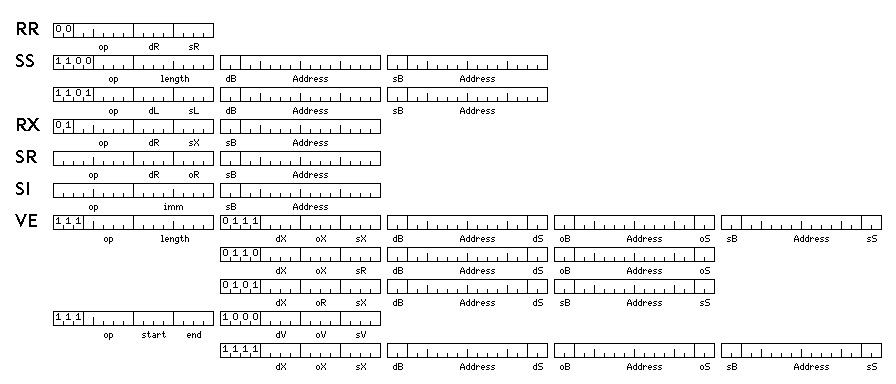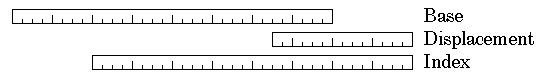[Next] [Up] [Previous] [Next Section] [Home] [Other]

# A Worked Example

To illustrate the design of a powerful computer, without running into excessive difficulties, it is necessary to avoid an overly complicated instruction set architecture (ISA). Thus, to keep complexity to a minimum, and yet present a full-power architecture, I have decided to model my example architecture very closely on that of the IBM System/360.The RR, SS, RX, SR, and SI instruction formats correspond to those of the System/360. The only difference is that the 16-bit address constants in an instruction consist of a two-bit base register field, and a 14-bit displacement field. This allows a base register to point to 16 kilobytes of memory rather than only 4 kilobytes of memory.

The values of this field indicate which register to use as the base register as follows:

```00: GR 12
01: GR 13
10: GR 14
11  GR 15
```

Note that two zero bits in this field do not indicate absolute addressing; the contents of a base register are always added to the address for those instructions where the effective address is actually used as a pointer to memory, and for the Load Effective Address instruction. Shift instructions will be an exception to this rule, however.

Placing a two-bit base register field in the 16-bit address, instead of attempting to move the base register field into the first 16 bits of the instruction, allows the SR format to work properly for the Load Multiple and Store Multiple instructions.

The VE instruction format is added to perform pipelined vector calculations as a basic part of the architecture.

Vector instructions are three-address instructions, with a source operand, and operand operand, and a destination operand. They perform the operation <source> op <operand> and store the result in <destination>.

There are two forms of vector instruction.

In the first form, a vector may be from 1 to 256 elements in length; the length field of the instruction contains the length of the vector minus one. The source and operand operands may be vectors in memory, or a floating-point register may be indicated which serves as a scalar operand, to be applied to each element of the other operand.

In the second form, a vector may be from 1 to 16 elements in length. All operands are vector operands; an operand can be in memory, or in one of sixteen vector registers. Each vector register consists of sixteen floating-point registers, numbered from 0 to 15. The start and end fields of the instruction indicate the range of floating-point registers within a vector register that are used in an operation. To be interpreted in this fashion, the contents of the start field must be less than or equal to those of the end field. In this case, vectors in memory will correspond in length to the range of registers indicated, and even if the starting register is not register 0, operation will start with the first element of the vector in memory that is addressed.

Alternatively, if the start field contains 14, and the end field contains a number between 0 and 7, then the least significant 16 bits contained in the general register indicated by the contents of the end field are used as a mask indicating on which elements of a 16-element vector the operation is performed. In this case, vectors in memory are treated as being exactly 16 elements in length, with elements skipped over in any part. If the start field contains 15, and the end field contains a number between 0 and 7, then a general register from 8 through 15 is used as the mask register.

For both forms of vector operation, as vector operations are only provided for aligned operands 32 bits or more in length, the last two bits of the displacement field will normally be zero in all cases. As a result, these fields are instead used for another purpose. If they are not both zero, they indicate a register containing a stride value, as follows:

```00: no stride
01: GR 1
10: GR 2
11: GR 3
```

A stride of 1 indicates that an area of memory corresponding to one item of the width of the type of number on which the instruction operates is skipped, and so on.

While 16-element vector registers are considerably shorter than the 64-element vector registers of the Cray I, they still allow vector calculations to take place without unnecessary memory accesses; the additional instruction fetch and decoding overhead is balanced by the reduced resource use and reduced overhead of context switching.

Address formation differs from that in the System/360 in several ways.In addition to the displacement field in an instruction being 14 bits long instead of 12 bits long, the contents of the base register used are shifted to the left eight bits before being added. This results in a 40-bit virtual address without a requirement to go into a 64-bit mode.

Also, unlike the IBM System/360, this system will, of course, use the IEEE 754 floating-point format as its native floating-point format.

It will have 16 general registers, each 32 bits in length, 16 floating-point registers, each 128 bits in length, and 16 vector registers, each composed of 16 floating-point registers, 128 bits in length.

The instructions that would be supported, and their opcodes, would be:

```RR format

00 LPER  Load Positive Extended Register
01 LNER  Load Negative Extended Register
02 LTER  Load and Test Extended Register
03 LCER  Load Complement Extended Register
04 HER   Halve Extended Register
05 BALR  Branch and Link Register
06 BCTR  Branch on Count Register
07 BCR   Branch on Condition Register
09 CER   Compare Extended Register
0B SER   Subtract Extended Register
0C MER   Multiply Extended Register
0D DER   Divide Extended Register
0E AUER  Add Unnormalized Extended Register
0F SUER  Subtract Unnormalized Extended Register

12 LTR   Load and Test Register
14 NR    AND Register
15 LCR   Logical Compare Register
16 OR    OR Register
17 XR    XOR Register
19 CR    Compare Register
1B SR    Subtract Register
1C MR    Multiply Register
1D DR    Divide Register
1F SLR   Subtract Logical Register

20 LPDR  Load Positive Double Register
21 LNDR  Load Negative Double Register
22 LTDR  Load and Test Double Register
23 LCDR  Load Complement Double Register
24 HDR   Halve Double Register
25 SVC   Supervisor Call

29 CDR   Compare Double Register
2B SDR   Subtract Double Register
2C MDR   Multiply Double Register
2D DDR   Divide Double Register

30 LPFR  Load Positive Floating Register
31 LNFR  Load Negative Floating Register
32 LTFR  Load and Test Floating Register
33 LCFR  Load Complement Floating Register
34 HFR   Halve Floating Register

39 CFR   Compare Floating Register
3B SFR   Subtract Floating Register
3C MFR   Multiply Floating Register
3D DFR   Divide Floating Register

RX Format

40 STH   Store Halfword
42 STC   Store Character
43 IC    Insert Character
44 XEC   Execute
46 BCT   Branch on Count
47 BC    Branch on Condition
49 CH    Compare Halfword
4B SH    Subtract Halfword
4C MH    Multiply Halfword
4D DH    Divide Halfword
4E CVD   Convert to Decimal
4F CVB   Convert to Binary

50 ST    Store

54 N     AND
55 CL    Compare Logical
56 O     OR
57 X     Exclusive OR
59 C     Compare
5B S     Subtract
5C M     Multiply
5D D     Divide
5F SL    Subtract Logical

60 STD   Store Double
61 CE    Compare Extended
63 SE    Subtract Extended
64 ME    Multiply Extended
65 DE    Divide Extended
67 SUE   Subtract Unnormalized Extended
69 CD    Compare Double
6B SD    Subtract Double
6C MD    Multiply Double
6D DD    Divide Double

6F STE   Store Extended

70 STF   Store Floating

79 CF    Compare Floating
7B SF    Subtract Floating
7C MF    Multiply Floating
7D DF    Divide Floating

RS, SI Format

82 LPSW  Load Program Status Word

86 BXH   Branch on Index High
87 BXLE  Branch on Index Low or Equal
88 SRSL  Shift Right Single Logical
89 SLSL  Shift Left Single Logical
8A SRS   Shift Right Single
8B SLS   Shift Left Single
8C SRDL  Shift Right Double Logical
8D SLDL  Shift Left Double Logical
8E SRD   Shift Right Double
8F SLD   Shift Left Double

90 STM   Store Multiple
92 MVI   Move Immediate

94 NI    AND Immediate
95 CLI   Compare Logical Immediate
96 OI    OR Immediate
97 XI    XOR Immediate

SS Format

C1 MVN   Move Numeric
C2 MVC   Move Character
C3 MVZ   Move Zone
C4 NC    AND Character
C5 CLC   Compare Logical Character
C6 OC    OR Character
C7 XC    XOR Character

CC TR    Translate
CD TRT   Translate and Test
CE ED    Edit
CF EDMK  Edit and Mark

D1 MVO   Move With Offset
D2 P     Pack
D3 U     Unpack

D9 CP    Compare Packed
DB SP    Subtract Packed
DC MP    Multiply Packed
DD DP    Divide Packed

VE Format

E0 STDV  Store Double Vector
E1 CEV   Compare Extended Vector
E3 SEV   Subtract Extended Vector
E4 MEV   Multiply Extended Vector
E5 DEV   Divide Extended Vector
E6 AUEV  Add Unnormalized Extended Vector
E7 SUEV  Subtract Unnormalized Extended Vector
E9 CDV   Compare Double Vector
EB SDV   Subtract Double Vector
EC MDV   Multiply Double Vector
ED DDV   Divide Double Vector

EF STEV  Store Extended Vector

F0 STFV  Store Floating Vector